# Understanding Matplotlib With Examples

After you have finished with Numpy and Pandas, you might be interested in playing with visualizing for static and interactive visuals in Python. Thus Matplotllib can be used to deliver or to abstract visuals of data upon required output so that the user can understand it better with both data graphically and in a visual manner.

Matplotlib can be installed using pip, as

``````python -m pip install -U pip
python -m pip install -U matplotlib``````

or you can install it on Anaconda main channel by

``conda install matplotlib``

also, you may install it via the conda-forage community channel

``conda install -c conda-forge matplotlib``

For Linux Package Manager

For Ubuntu or Debian :

``sudo apt-get install python3-matplotlib``

For Arch :

``sudo pacman -S python-matplotlib``

For RedHat :

``sudo yum install python3-matplotlib``

## Line Plot using Matplotib

To build a line plot using Matplotib

``````# importing matplotlib module
from matplotlib import pyplot as plt

# x-axis values stored in A
A = [25, 74, 56, 75, 50]

# Y-axis values stored in B
B = [20, 50, 38, 39, 40]

# Function to plot
plt.plot(A,B)

# function to show the plot
plt.show()
``````

Output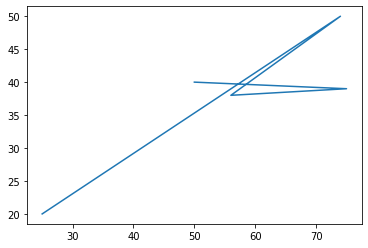## Histogram using Matplotib

To build a Histogram using Matplotlib

``````# importing matplotlib module
from matplotlib import pyplot as plt

# Y-axis values stored in B
B = [22, 40, 55, 32, 20]

# Function to plot histogram
plt.hist(B)

# Function to show the plot
plt.show()
``````

Output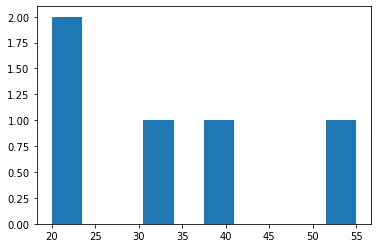## Scatter Plot using Matplotib

To build a Scatter Plot using Matplotlib

``````# importing matplotlib module
from matplotlib import pyplot as plt

# x-axis values stored in A
A = [6,8,7,2,9]

# Y-axis values stored in B
B = [12,15,8,9,10]

# Function to plot scatter
plt.scatter(A, B)

# function to show the plot
plt.show()
``````

Output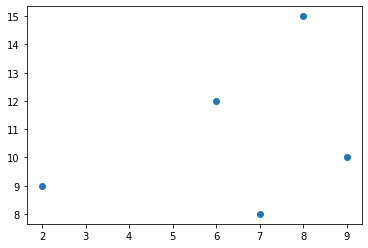## BarPlot using Matplotib

To build a BarPlot using MatplotLib

``````# importing matplotlib module
from matplotlib import pyplot as plt

# x-axis values stored in A
A = [7,8,6,9,3]

# Y-axis values stored in B
B = [15, 25, 18, 12, 23]

# Function to plot the bar
plt.bar(A,B)

# function to show the plot
plt.show()``````

Output## SinWave using Matplotib

To build a SinWave using Matplotlib

``````from matplotlib import pyplot as plt
import numpy as np
import math
x = np.arange(0, math.pi*2, 0.045)
y = np.sin(x)
plt.plot(x,y)
plt.xlabel("Angle")
plt.ylabel("Sine")
plt.title('SineWave')
plt.show()``````

Output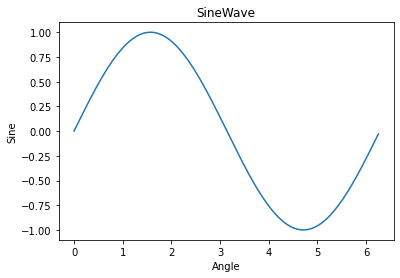## 3D Surface Plot using Matplotib

To build a 3D Surface Plot using Matplotlib

``````from mpl_toolkits import mplot3d
import numpy as np
import matplotlib.pyplot as plt
A = np.outer(np.linspace(-1.1, 1.1, 100), np.ones(100))
B = A.copy().T
C = np.cos(A ** 2 + B ** 2)
fig = plt.figure()
ax = plt.axes(projection='3d')
ax.plot_surface(A, B, C,cmap='viridis', edgecolor='none')
ax.set_title('SurfacePlot using MatplotLib')
plt.show()``````

Output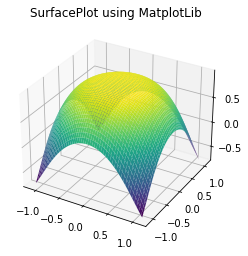The above were a few examples of how one can implement matplotlib, one can have a good amount of grip upon practice. Thus patience, practice, and time need to be invested while learning. Till then keep enjoying and keep coding.